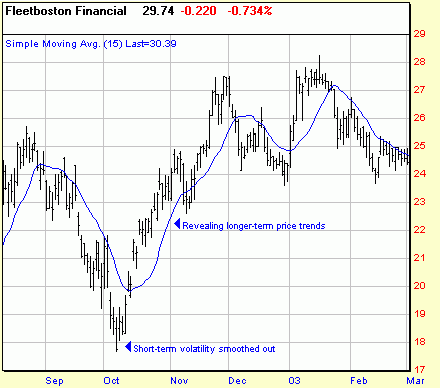# Simple Moving Average

A Simple Moving Average (SMA) is calculated by adding the closing price of a security for a number of time periods and then dividing this total by the number of time periods. This illustrates the average price of the issue over a set number of periods. The moving average smooths the data series and makes it easier to spot trends. See also: Exponential Moving Average

Related Articles: Understanding Moving AveragesChart courtesy of Prophet Financial Systems